Question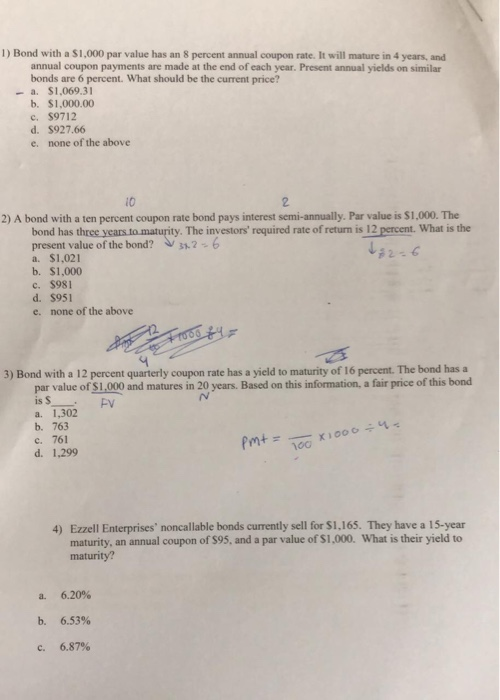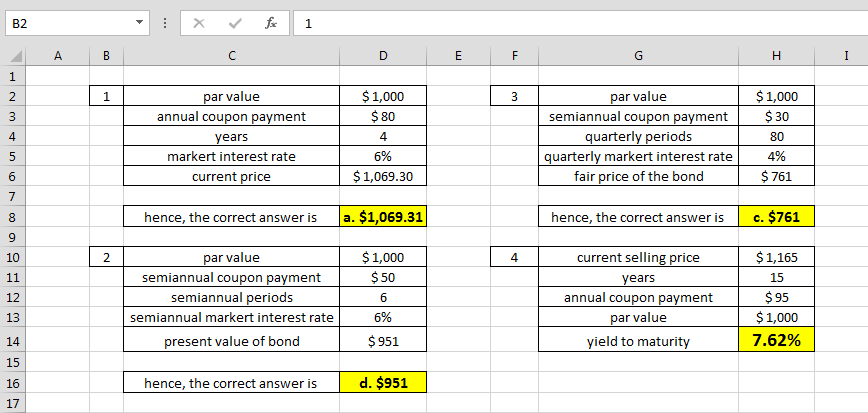for formulas and calculations, refer to the image below -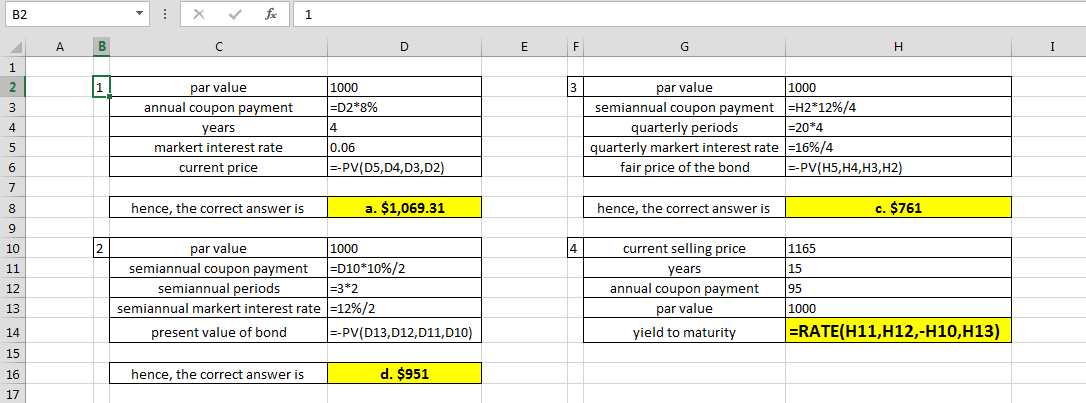#### Earn Coins

Coins can be redeemed for fabulous gifts.

Similar Homework Help Questions
• ### A bond with a par value of \$1,000 and an annual coupon rate of 9.5% has...

A bond with a par value of \$1,000 and an annual coupon rate of 9.5% has a yield to maturity of 6.4%. If the bond has 11 years to maturity, what is its current yield?

• ### A bond has a par value of \$1,000, a current yield of 8.15 percent, and semiannual coupon payments. The bond is quoted at 103.51. What is the coupon rate of the bond?

1. A bond has a par value of \$1,000, a current yield of 8.15 percent, and semiannual coupon payments. The bond is quoted at 103.51. What is the coupon rate of the bond?2. Kasey Corp. has a bond outstanding with a coupon rate of 5.94 percent and semiannual payments. The bond has a yield to maturity of 5.1 percent, a par value of \$2,000, and matures in 20 years. What is the quoted price of the bond?3. A bond with...

• ### A semi-annual coupon bond has a 6 percent coupon rate, a \$1,000 face value, a current...

A semi-annual coupon bond has a 6 percent coupon rate, a \$1,000 face value, a current value of \$1,036.09, and 3 years until the first call date. What is the call price if the yield to call is 6.5 percent? A STRIPS has a yield to maturity of 6.2 percent, a par value of \$25,000, and a time to maturity of 10 years. What is the price

• ### 2. A coupon bond pays annual int coupon rate of 10%, and has a yield to...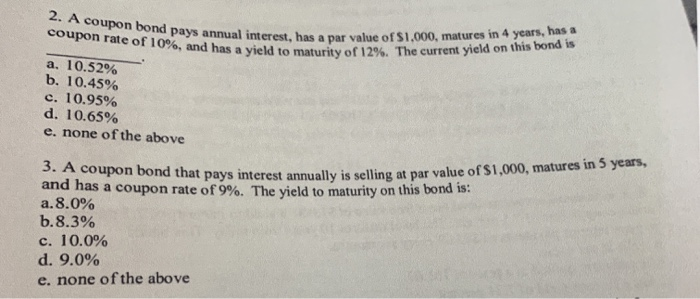2. A coupon bond pays annual int coupon rate of 10%, and has a yield to maturity of annual interest, has a par value of \$1.000, matures in 4 years, has a ed to maturity of 12%. The current yield on this bond is a. 10.52% b. 10.45% c. 10.95% d. 10.65% e. none of the above 3. A coupon bond that pays interest annually is selling op and has a coupon rate of 9%. The yield to maturity on...

• ### Today, a bond has a coupon rate of 8.86 percent, par value of 1,000 dollars, YTM...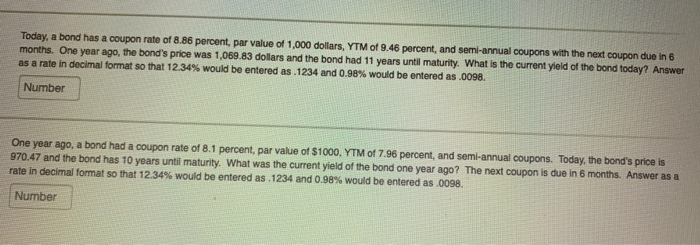Today, a bond has a coupon rate of 8.86 percent, par value of 1,000 dollars, YTM of 9.46 percent, and semi-annual coupons with the next coupon due in 6 months. One year ago, the bond's price was 1,069.83 dollars and the bond had 11 years until maturity. What is the current yield of the bond today? Answer as a rate in decimal format so that 12.34% would be entered as.1234 and 0.98% would be entered as .0098. Number One year...

• ### A bond that matures in 12 years has a \$1,000 par value. The annual coupon interest rate is 11 percent and the​ market's...

A bond that matures in 12 years has a \$1,000 par value. The annual coupon interest rate is 11 percent and the​ market's required yield to maturity on a​ comparable-risk bond is 17 percent. What would be the value of this bond if it paid interest​ annually_____? What would be the value of this bond if it paid interest​ semiannually_____? a.  The value of this bond if it paid interest annually would be ​\$nothingm. ​(Round to the nearest​ cent.)

• ### A bond that matures in 17 years has a ​\$1 000 par value. The annual coupon...

A bond that matures in 17 years has a ​\$1 000 par value. The annual coupon interest rate is 14 percent and the​ market's required yield to maturity on a​ comparable-risk bond is 17 percent. What would be the value of this bond if it paid interest​ annually? ______ What would be the value of this bond if it paid interest​ semiannually? _______

• ### Gugenheim, Inc., has a bond outstanding with a coupon rate of 5.5 percent and annual payments....

Gugenheim, Inc., has a bond outstanding with a coupon rate of 5.5 percent and annual payments. The yield to maturity is 6.7 percent and the bond matures in 11 years. What is the market price if the bond has a par value of \$2,000?

• ### Analyze the 20-year, 8% coupon rate (annual payment), \$1,000 par value bond. The bond currently sells...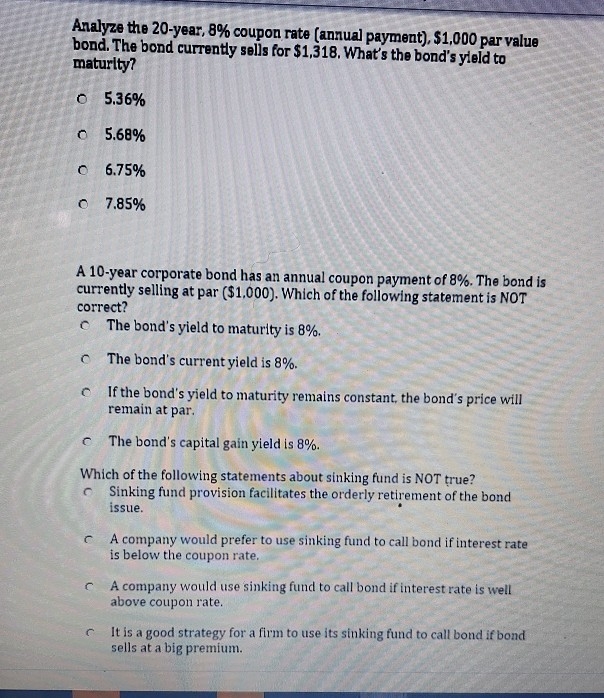Analyze the 20-year, 8% coupon rate (annual payment), \$1,000 par value bond. The bond currently sells for \$1,318. What's the bond's yield to maturity? o o 5.36% 5.68% o 6.75% o 7.85% A 10-year corporate bond has an annual coupon payment of 8%. The bond is currently selling at par (\$1.000). Which of the following statement is NOT correct? The bond's yield to maturity is 8%. The bond's current yield is 8%. If the bond's yield to maturity remains constant,...

• ### Consider a coupon bond that has a par value of\$900 and a coupon rate of 8%....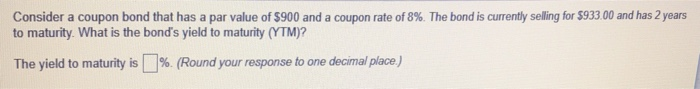Consider a coupon bond that has a par value of\$900 and a coupon rate of 8%. The bond is currently selling for\$933 00 and has 2 years to maturity. What is the bond's yield to maturity (YTM)? The yield to maturity is 1% Roundyour response to one deama/place